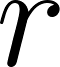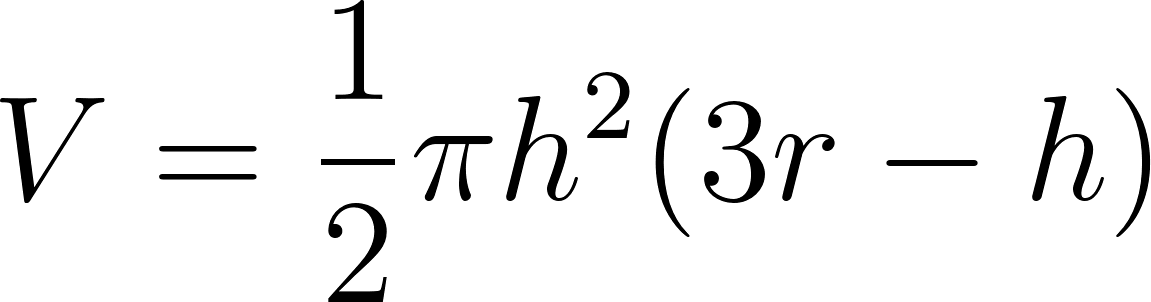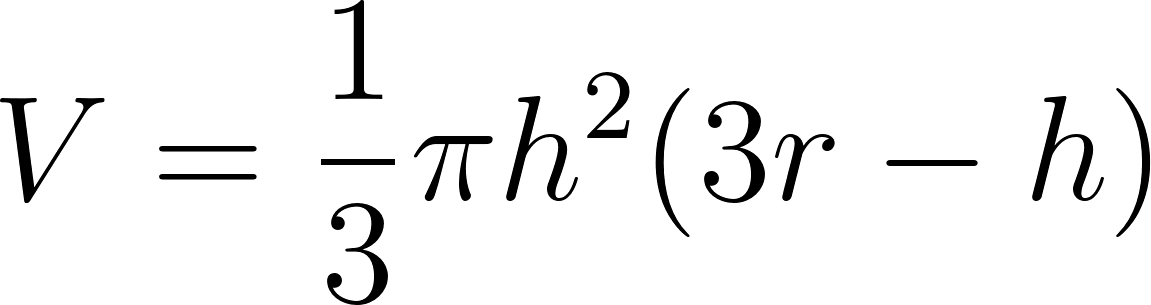×
Get Full Access to Calculus: Early Transcendentals - 1 Edition - Chapter 1.2 - Problem 59e
Get Full Access to Calculus: Early Transcendentals - 1 Edition - Chapter 1.2 - Problem 59e

×

# Functions from eometry A single slice through a sphere ofISBN: 9780321570567 2

## Solution for problem 59E Chapter 1.2

Calculus: Early Transcendentals | 1st Edition

• Textbook Solutions
• 2901 Step-by-step solutions solved by professors and subject experts
• Get 24/7 help from StudySoup virtual teaching assistantsCalculus: Early Transcendentals | 1st Edition

4 5 1 284 Reviews
12
4
Problem 59E

Functions and geometry: A single slice through a sphere of radiusproduces a cap of the Sphere. If the thickness of the cap is, then its volume is. Graph the volume as a function offor a sphere of radius 1. For what values ofDoes this function make sense?

Step-by-Step Solution:

Step 1 of 4

Given,

A single slice through a sphere of radiusproduces a cap of the Sphere.

The thickness of the cap is, then its volume is ,.

Step 2 of 4

Step 3 of 4

##### ISBN: 9780321570567

This full solution covers the following key subjects: sphere, function, Volume, cap, radius. This expansive textbook survival guide covers 112 chapters, and 7700 solutions. This textbook survival guide was created for the textbook: Calculus: Early Transcendentals, edition: 1. The answer to “?Functions and geometry: A single slice through a sphere of radius produces a cap of the Sphere. If the thickness of the cap is , then its volume is . Graph the volume as a function of for a sphere of radius 1. For what values of Does this function make sense?” is broken down into a number of easy to follow steps, and 52 words. Since the solution to 59E from 1.2 chapter was answered, more than 435 students have viewed the full step-by-step answer. The full step-by-step solution to problem: 59E from chapter: 1.2 was answered by , our top Calculus solution expert on 03/03/17, 03:45PM. Calculus: Early Transcendentals was written by and is associated to the ISBN: 9780321570567.

## Discover and learn what students are asking

Calculus: Early Transcendental Functions : Area and Arc Length in Polar Coordinates
?In Exercises 5-16, find the area of the region. One petal of $$r=4 \sin 3 \theta$$

Calculus: Early Transcendental Functions : The Cross Product of Two Vectors in Space
?In Exercises 1-6, find the cross product of the unit vectors and sketch your result. $$\mathbf{k} \times \mathbf{j}$$

Calculus: Early Transcendental Functions : Conservative Vector Fields and Independence of Path
?In Exercises 1 - 4, show that the value of $$\int_{C} F \cdot d r$$ is the same for each parametric representation of C. \(\mathbf{F}(x, y)=y

Statistics: Informed Decisions Using Data : Applications of the Normal Distribution
?The notation z? is the z-score such that the area under the standard normal curve to the right of z? is _______ .

Statistics: Informed Decisions Using Data : The Normal Approximation to the Binomial Probability Distribution
?In Problems 5–14, a discrete random variable is given. Assume the probability of the random variable will be approximated using the normal distributio

Statistics: Informed Decisions Using Data : Estimating a Population Standard Deviation
?True or False: The shape of the chi-square distribution depends on its degrees of freedom.

Unlock Textbook Solution